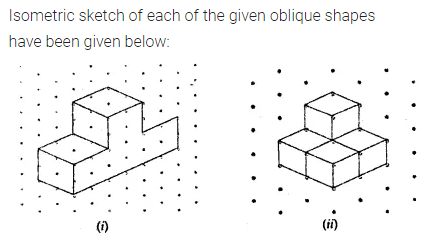# ML Aggarwal Class 7 Solutions for ICSE Maths Chapter 15 Visualising Solid Shapes Ex 15.2

## ML Aggarwal Class 7 Solutions for ICSE Maths Chapter 15 Visualising Solid Shapes Ex 15.2

Question 1.
The dimensions of a cuboid are 5 cm, 3 cm, and 2 cm. Draw three different isometric sketches of this cuboid with height
(i) 2 cm
(ii) 3 cm
(iii) 5 cm
Solution: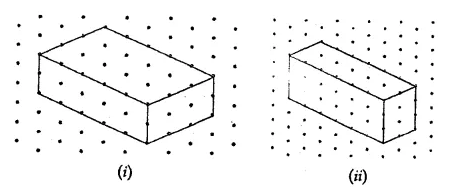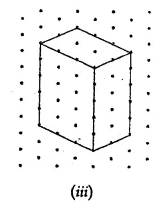Question 2.
Give (i) an oblique sketch (ii) an isometric sketch for each of the following:
(a) A cube is with an edge 4 cm long.
(b) A cuboid of length 6 cm, breadth 4 cm, and height 3 cm.
Solution:Question 3.
The adjoining figure shows an isometric sketch of a 3-D shape. Draw an oblique sketch that matches this sketch.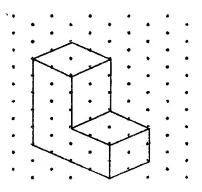Solution:Question 4.
Draw an isometric sketch of each of the following oblique shapes: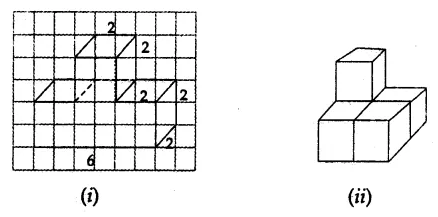Solution: